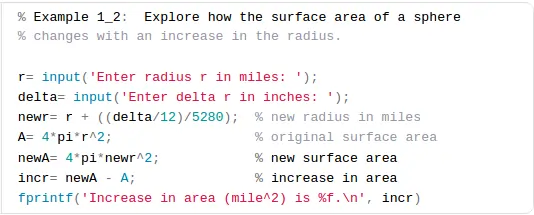# Check Out The MATLAB Assignment Samples | EssayCorpThis MATLAB assignment samples series is aimed to help students to begin with MATLAB Programming. For advanced and complicated problem solving, these basics are helpful to develop building block. Essaycorp help students to solve complex MATLAB assignment.

In this series , these problems are solved

1. Calculation of area of a sphere and calculate differential changes ( relative changes in area of sphere with change in radius)
2. For a given quadratic expression , find the minimum value and RHS and LHS limits
1. Calculate Area of Sphere
```% Example 1_1:  Compute surface area of a sphere
% A: surface area of the sphere
% r: radius of the sphere

A= 4*3.14159*r*r;
fprintf('Surface area is %f!
', A)```
2. How the surface area of a sphere % changes with an increase in the radius
```% Example 1_2:  Explore how the surface area of a sphere
% changes with an increase in the radius.

r= input('Enter radius r in miles: ');
delta= input('Enter delta r in inches: ');
newr= r + ((delta/12)/5280);  % new radius in miles
A= 4*pi*r'2;                  % original surface area
newA= 4*pi*newr'2;            % new surface area
incr= newA - A;               % increase in area
fprintf('Increase in area (mile'2) is %f.
', incr)```
3. Find min of q(x)=x'2+bx+c given b,c and random L,R
```% Find min of q(x)=x'2+bx+c given b,c and random L,R

b= 2;  c= -1.5;     % coefficients of q(x)
L= rand*10 - 8;     % left end of interval, random in (-8,2)
R= L + 1 + rand*10; % right end of interval, random in (-7,13)

xc = -b/2;  % critical value

if (L<=xc && xc<=R)
% min is at xc
qMin= xc'2 + b*xc + c;
else
% min is at L or R
if xc < L
qMin= L'2 + b*L + c;
else
qMin= R'2 + b*R + c;
end
end

disp('Quadratic function q(x) = x'2 + bx + c')
fprintf('    b=%.2f    c=%.2f
', b,c)
fprintf('The minimum value in [%.2f,%.2f] is %.4f
', L,R,qMin)```

We Provide MATLAB assignment help. To get help from MATLAB expert, fill the instant quote.

MATLAB assignment help, MATLAB assignment,